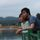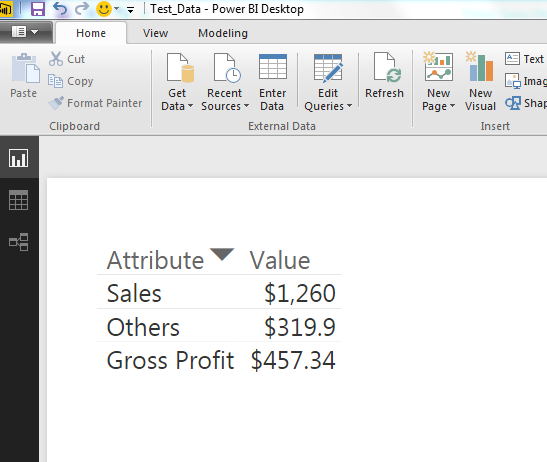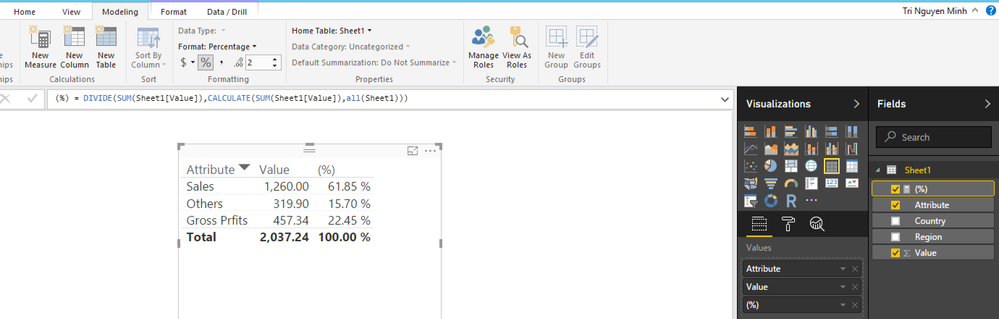cancel
Showing results for
Did you mean:Frequent Visitor

## How to use a calculated measure in a matrix/table visualisation

I have a database where I have the country, region etc in columns, and in another column attributes like Sales, Gross Profit, other costs etc and in the last column the values. I am trying to calculate the Gross Profit as % of Sales in a calculated measure and use this measure in the Matrix / Table visualisation. In the table I have in the rows Sales, Gross Profit, other costs and so on (from attributes column of database) and in columns I have the values for these. I want to add Gross profit % of Sales in the rows and in the column the value of this. When I try to do this I get the calculated measure on a separate column. Any help appreciated.

5 REPLIES 5Memorable Member

Oh, sorry, i posted wrong topic.Frequent VisitorThanks. But the data I have is as above. and the visualisation table is as below:and in the above visualisation table I need to add the calculated measure (gross profit / Sales) which will be 36.3%Memorable Member

Hi @iqbalck,

I think with ALL method you could calculate sum of total:

`(%) = DIVIDE(SUM(Sheet1[Value]),CALCULATE(SUM(Sheet1[Value]),all(Sheet1)))`Hope this is what you are expecting.Frequent Visitor

No, I am trying to insert the calculated measure below the Gross Profit. You have inserted the calculation on the new column instead of the row. And also the calculation is not required as sum of total.Microsoft

Hi @iqbalck

Based on your description, it seems that you want to create a table/Matrix visual as below. If that is the case, it is not possible.In your current table visual, each row is consist of values of Attribute field and Value field , the measure you create is just a calculated value based on the values of Attribute field and Value field, you can create another visual to host the measure.

Thanks,
Lydia Zhang

Community Support Team _ Lydia Zhang
If this post helps, then please consider Accept it as the solution to help the other members find it more quickly.